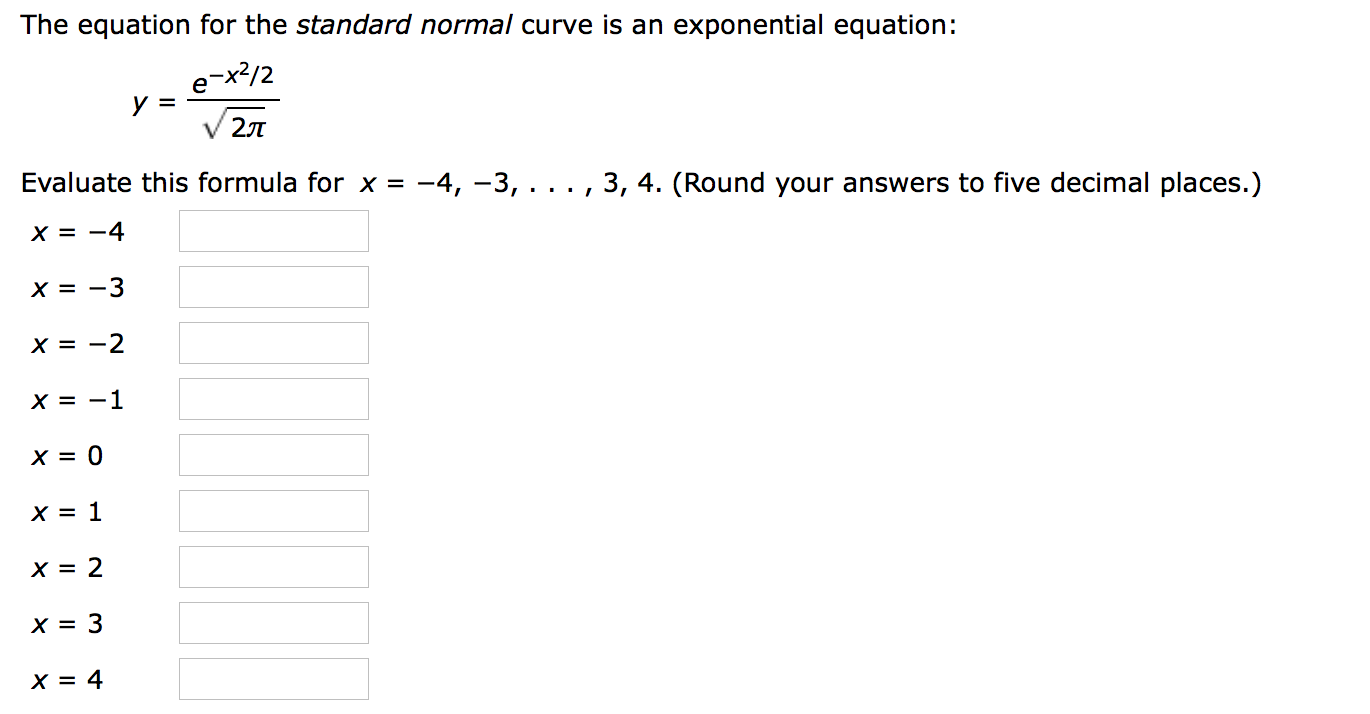# The equation for the standard normal curve is an exponential equation:е -x/2у %32JTEvaluate this formula for x-4, -3, . . . , 3, 4. (Round your answers to five decimal places.)X = -4X -3х3D —2X= -1х—D 0X= 1X= 2X= 3X = 4

Question
2 viewshelp_outlineImage TranscriptioncloseThe equation for the standard normal curve is an exponential equation: е -x/2 у %3 2JT Evaluate this formula for x -4, -3, . . . , 3, 4. (Round your answers to five decimal places.) X = -4 X -3 х3D —2 X= -1 х—D 0 X= 1 X= 2 X= 3 X = 4 fullscreen
check_circle

Step 1

The provided equation is:

Step 2

Plug, x = -4 in the provided equation as:

Step 3

Substitute, x= -3 in the ...

### Want to see the full answer?

See Solution

#### Want to see this answer and more?

Solutions are written by subject experts who are available 24/7. Questions are typically answered within 1 hour.*

See Solution
*Response times may vary by subject and question.
Tagged in

### Other